# Electronics and Communication Engineering - Electromagnetic Field Theory

26.

Consider a loss less antenna with a directive gain of + 6 dB. If 1 mW of power is fed to it the total power radiated by the antenna will be

 A. 4 mW B. 1 mW C. 7 mW D. 1/4 mW

Explanation:

6 dB = 10 log10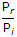= 100.63.98

Pr = 3.98 mW.

27.

A uniform plane wave is one in which

 A.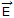x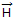= 0 B..C.andare perpendicular D.andlie in a plane

Explanation:

For uniform plane wave, E and H must be in a plane but perpendicular to each other.

28.

The electric field of the incident wave is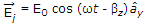, where ω = 3 x 109p and β = 10 p. The electric field of the transmitted wave Et is given by

 A.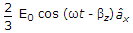B.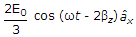C.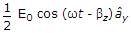D.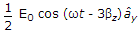Explanation:

In, question, it is not mentioned, about medium; dielectric etc.

Ei = Et + ErEt = ErEt =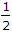Ei.

29.

A short circuited stub is shunt connected to a transmission line as shown in the figure is, if z0 = 500 ohms, the admittance y seen at the function of the stub and the transmission line is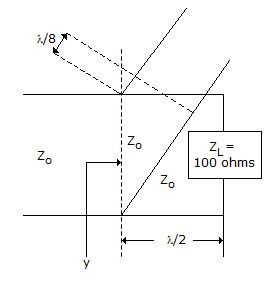A. (0.01 - j 0.02) mho B. (0.02 - j 0.01) mho C. (0.04 - j 0.02) mho D. (0.02 + j 0) mho

Explanation: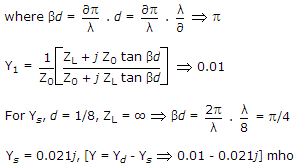30.

Which of the following should dominant wave have?

 A. Lowest cut off frequency B. Highest cut off frequency C. No attenuation D. No phase shift